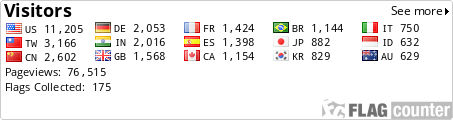About me | pbdR Tech | HPSC | Phyloclustering | R_note |

 R_note -- The Exploration of Statistical Software R (²Î­p³nÅé R ²`«×¾úÀI)
Reference
MS Windows

Batch jobs
Function
Data/MySQL
Plot
String/Parse

Remark Lines
Classes/S3
S4 Methods
Batch more
Environment

Good Coding
Fast Loop
LAM/MPI/Rmpi
Recursion

PHP Call R
Basic C
R Call Fortran/C
R Call GSL
C Call R API
C Call R Objects
Standalone

Make Packages
C Pointer
Debug

Section: Plot

• Plot
The `plot()` function is defined as,

 ``` plot(x, y, xlim=range(x), ylim=range(y), type="p", main, xlab, ylab, ...) ```

In file "plot_1.r" will be a simple X-Y plot,

 ``` # File name: plot_1.r data(cars) plot(cars) plot(cars, type="l") ```

In file "plot_2.r" will be a user defined X-Y plot,

 ``` # File name: plot_2.r data(cars) windows() plot(cars, axes = FALSE, xlab = "", ylab = "") lines(cars) points(cars, pch = 23, cex = 1.5, col = 2) axis(1, lwd = 2, lty = 2) axis(2, col = 3, las = 2) box(lty = 3) title(main = "cars", xlab = "x-axis", ylab = "y-axis") legend(5, 100, "cars", lty = 1) dev.list() dev.cur() dev.off() ```

• Layout
Here, in file "plot_3.r" put the 4 plots in the same graph for simple layout,

 ``` # File name: plot_3.r data(cars) par(mfrow = c(2, 2)) plot(cars, main = "Cars") hist(cars[, 1], xlab = "speed", main = "Histogram of speed") hist(cars[, 2], xlab = "dist", nclass = 10, main = "Histogram of dist") plot(density(cars[, 2]), main = "Density of dist") ```

And, the file "plot_4.r" put the 4 plots in the same graph for user defined layout,

 ``` # File name: plot_4.r data(cars) layout(matrix(c(2, 4, 1, 3), nrow = 2, byrow = TRUE), c(2, 1), c(1, 2)) par(mar = c(3, 3, 1, 1)) plot(cars, xlab = "", ylab = "") par(mar = c(3, 3, 1, 1)) hist(cars[, 1], main = "", xlab = "", ylab = "") par(mar = c(0, 3, 1, 1)) hist(cars[, 2], nclass = 10, main = "", xlab = "", ylab = "") par(mar = c(3, 3, 1, 1)) plot(density(cars[, 2]), main = "", xlab = "", ylab = "") ```

• Save
The bitmap function require a command `gs`, a software GhostScript, to transform .ps image file to another type.
About plot graph save function has follows,

 ``` postscript(file = ifelse(onefile, "Rplots.ps", "Rplot%03d.ps"), onefile = TRUE, paper, family, encoding, bg, fg, width, height, horizontal, pointsize, pagecentre, print.it, command, title = "R Graphics Output") ``` ``` bitmap(file, type = "png256", height = 6, width = 6, res = 72, pointsize, ...) ``` ``` bmp(filename = "Rplot%03d.bmp", width = 480, height = 480, pointsize = 12, bg = "white") jpeg(filename = "Rplot%03d.jpg", width = 480, height = 480, pointsize = 12, quality = 75, bg = "white") png(filename = "Rplot%03d.png", width = 480, height = 480, pointsize = 12, bg = "white") ```

The file "plot_5.r" will save the graph in "plot_4.r" as a jpeg file "plot_5.png",

 ``` # File name: plot_5.r data(cars) bitmap("plot_5.png", height = 8, width = 8) # In Windows, use bmp() or jpeg() layout(matrix(c(2, 4, 1, 3), nrow = 2, byrow = TRUE), c(2, 1), c(1, 2)) par(mar = c(3, 3, 1, 1)) plot(cars, xlab = "", ylab = "") par(mar = c(3, 3, 1, 1)) hist(cars[, 1], main = "", xlab = "", ylab = "") par(mar = c(0, 3, 1, 1)) hist(cars[, 2], nclass = 10, main = "", xlab = "", ylab = "") par(mar = c(3, 3, 1, 1)) plot(density(cars[, 2]), main = "", xlab = "", ylab = "") dev.off() ```

• More
see Package `grid`.

 [ Go to top ]

 Maintained: Wei-Chen Chen E-Mail: wccsnow @ gmail.com Last Revised: Dec 12 2016, 09:44 (CST Taipei, Taiwan) Created: Oct 06 2003Best Resolution IE6.0 1280x1024 small font